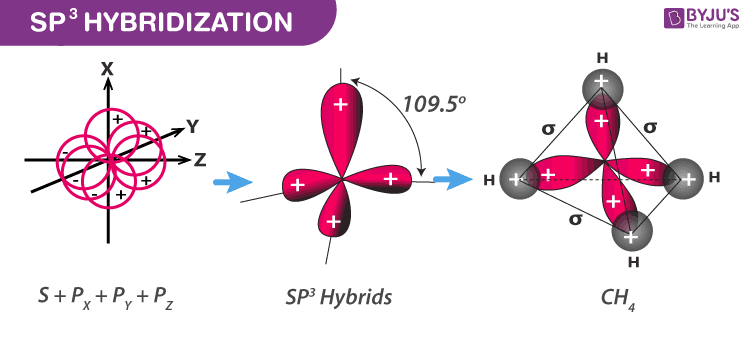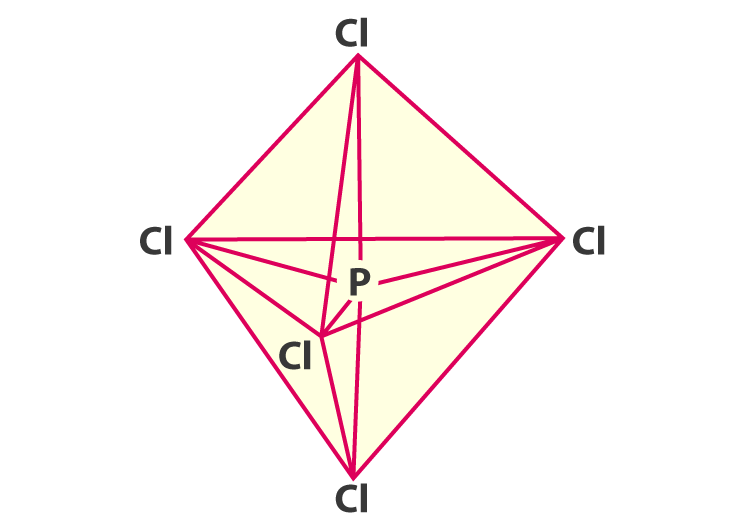# PCl5 Hybridization

## PCl5 Hybridization Introduction

The elements that are present in the third period comprise d orbitals along with s and p orbitals. The energy of the 3d orbitals is close to the energy of 3s as well as 3p orbitals. The energy of 3d orbitals is also equivalent to 4s as well as 4p orbitals. As a result, the hybridization including either 3s, 3p and 3d or 3d, 4s, and 4p is feasible. Due to the difference in energies of 3p and 4s orbitals, no hybridization including 3p, 3d, and 4s orbitals is possible.

The important hybridizations including s, p and d orbitals are briefly discussed below:

 Shape of molecule Type of hybridization Atomic orbitals Example Square planar dsp2 d+s+p(2) [Ni(CN)4]2– Trigonal bipyramidal sp3d s+p(3)+d PCl5 Square pyramidal sp3d2 s+p(3)+d(2) BrFl5 Octahedral Sp3d, d2sp3 s+p(3)+d(2), d(2)+s+p(3) [CrF6]3–, [Co(NH3)6]3+

## How is PCl5 formed?

The excited state and ground state of outer electronic configurations for Z =15 (phosphorus) is represented as below.sp3d hybridization

The five orbitals viz 1s, 3p, and 1d orbitals are free for hybridization. Therefore, it can obtain a set of 5sp3d hybrid orbitals directed to the 5 corners of a trigonal bipyramidal (VSEPR theory). The below diagram will help you depict easily.hybridization of pcl5

It is prominent that all the bond angles in trigonal bipyramidal geometry are not identical. In PCl5 the 5sp3d orbitals of phosphorus overlap with p orbitals of chlorine atoms. The p orbitals are singly occupied. Together they form 5 P–Cl sigma bonds.

### Types of bonds formed during the PCl5 hybridization-

Equatorial bonds: 3 P–Cl bond which lies in one plane to make an angle with each other. The angle made between them is 120°.

Axial bonds: 2 P–Cl bonds where one lies above the equatorial plane and the other below the plane to make an angle with the plane. The angle made with the plane 90°.

Since the axial bond pairs agonize more repulsive interaction from the equatorial bond pairs, the axial bonds tend to be slightly longer. Therefore, it makes it slightly weaker than the equatorial bonds resulting in obtaining more reactive PCl5 molecule.

To learn more about the hybridization of other atomic orbitals from the expert faculties register to BYJU’S now!